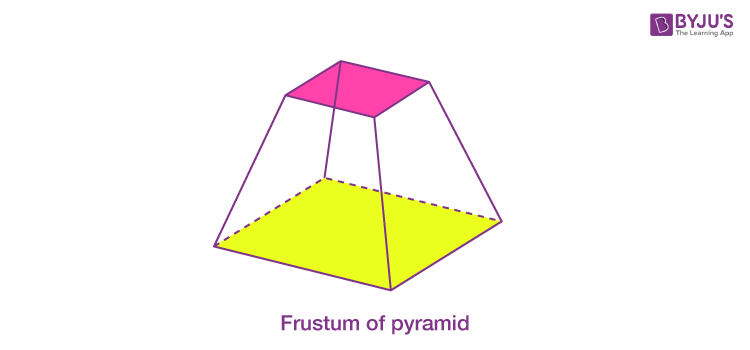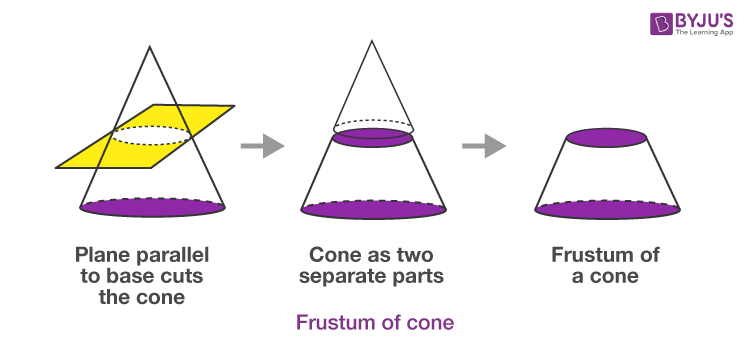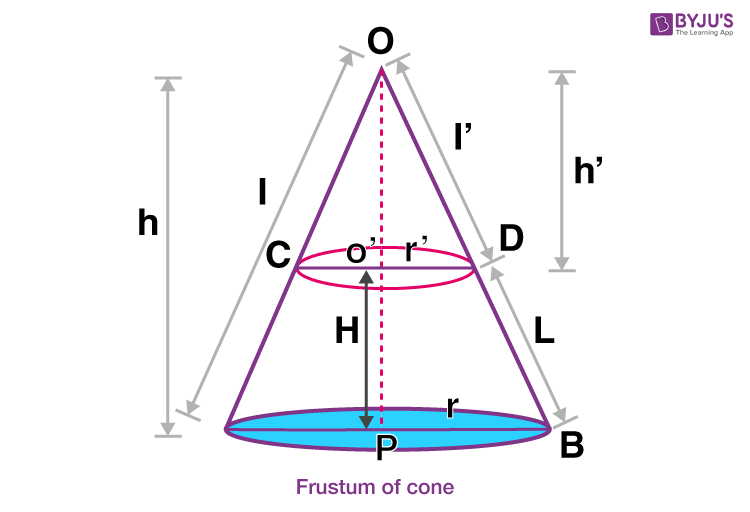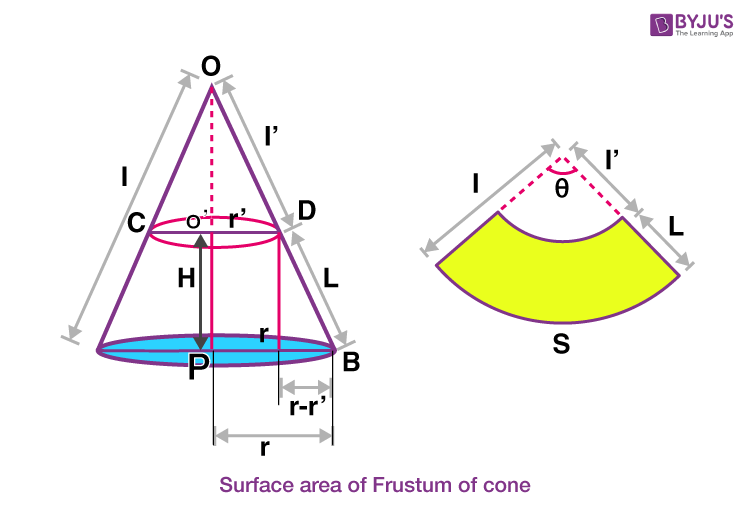# Frustum Of A Cone

Frustum of cone is the part of cone when it is cut by a plane into two parts. The upper part of cone remains same in shape but the bottom part makes a frustum. To get this part of the right circular cone we have to slice it horizontally or parallel to the base. Both pieces have different volumes and areas. Learn Volume Of Cone here.Frustum is a Latin word which means ‘piece cut off’. When a solid (generally a cone or a pyramid) is cut in such a manner that base of the solid and the plane cutting the solid are parallel to each other, part of solid which remains between the parallel cutting plane and the base is known as frustum of that solid.

To visualize a frustum properly, consider an ice-cream cone which is completely filled with ice-cream. When the cone is cut in a manner as shown in the figure, the section left between the base and parallel plane is the frustum of a cone.## Volume of Frustum Cone

Let us learn here how to find the volume of frustum of a cone with the help of diagram given. This formula will also help to find the volume of frustum of a pyramid which is shaped in the form of cone.In the cone given above, the frustum can be considered as the difference of two right circular cones.

Let the larger cone which has a height equal to “h” units, slant height as “l” units and radius as “r” units be named as cone 1 and the smaller right circular cone be named as cone 2 whose height is given as h’ units, radius as r’ units and the slant height as l’ units.

The height of frustum is”H” units and its slant height is “L” units.

$$\begin{array}{l}The\ volume\ of\ right\ circular\ cone\ 1 = \frac{1}{3}~ \pi r^{2} h\end{array}$$

Similarly,

$$\begin{array}{l}The\ volume\ of\ right\ circular\ cone\ 2 = \frac{1}{3}~ \pi r’^2 h'\end{array}$$

Therefore, the volume of the frustum of cone can be given as:

$$\begin{array}{l}Volume\ of\ frustum\ of\ cone\ V = \frac{1}{3}~ \pi r^2~ h~-~\frac{1}{3}~ \pi r’^2 h'\end{array}$$

$$\begin{array}{l}Volume\ of\ frustum,\ V = \frac{1}{3}~ \pi (r^2 ~h~-~r’^2 h’) …(1)\end{array}$$

From the figure given above, in the ∆OO’D and ∆OPB;

∠DOO’= ∠BOP(Common Angle)

$$\begin{array}{l}As\ \overleftrightarrow{CD}||\overleftrightarrow{AB}\ (Plane\ dividing\ the\ cone\ is\ parallel\ to\ the\ base)\end{array}$$

⇒∠O’DO = ∠PBO (Corresponding Angles)

Thus, ∆OO’D~∆OPD  (By AA criterion of similarity)

Hence, according to condition for similar triangles the ratio of corresponding sides must be equal:

$$\begin{array}{l}\Rightarrow \frac{h’}{h} = \frac{r’}{r} …(2)\end{array}$$

Substituting this value in equation (1),

$$\begin{array}{l}V = \frac{1}{3}~ \pi \left(r^2 \left(\frac{rh’}{r’}\right)~-~r’^2 h’\right)\end{array}$$

$$\begin{array}{l}\Rightarrow V = \frac{1}{3}~ \pi h’~ \left(\frac{r^3~-~r’^3}{r’}\right)…(3) \end{array}$$

As it can be seen from fig 3,

$$\begin{array}{l}h = H~+~h'\end{array}$$

Substituting this value in equation (2),

$$\begin{array}{l}\frac{h’}{H~+~h’} =\frac{r’}{r}\end{array}$$

$$\begin{array}{l}\Rightarrow \frac{h’}{H} = \frac{r’}{r~-~r’}\end{array}$$

$$\begin{array}{l}h’ = H\left(\frac{r’}{r~-~r’}\right)\end{array}$$

Substituting this value of h’ in equation (3), we get

$$\begin{array}{l}V=\frac{1}{3}\pi H(\frac{r{‘}}{r-r{‘}})(\frac{r^3-r{‘}^3}{r{‘}})\end{array}$$

$$\begin{array}{l}V=\frac{1}{3}\pi H(\frac{r-r{‘}}{r-r{‘}})({r^2+rr{‘}+r{‘}^2})\end{array}$$

$$\begin{array}{l}\Rightarrow V = \frac{1}{3}~ \pi H~(r^2~+~r’r~+~r’^2)\end{array}$$

This gives the required volume of frustum of cone.

## Surface Area of Frustum of Cone

Similarly, the surface area will also be the difference of the surface areas of both the cones.

Curved surface area of right circular cone 1 = πrl

Curved surface area of right circular cone 2 = πr’l’

Curved surface area of the frustum of cone = πrl – πr’l’

Also, from fig. 3 ,in the the ∆OAB and ∆OCD;

∠AOB = ∠COD           (Common Angle)

$$\begin{array}{l}As\ \overleftrightarrow{CD} || \overleftrightarrow{AB} \ (Plane\ dividing\ the\ cone\ is\ parallel\ to\ the\ base)\end{array}$$

⇒ ∠OCD = ∠OAB (Corresponding Angles)

Thus, ΔOAB~ΔOCD (By AA criterion of similarity)

Lateral surface area of frustum of the cone is the difference of the areas of sector of circles (s and  s′) with radii r and r′ and common central angle θ as shown in the figure.From fig. 3 as the ΔOO′D ~  ΔOPB therefore:

$$\begin{array}{l}\Rightarrow \frac{l’}{l} = \frac{r’}{r}…(4)\end{array}$$

Also from the figure given above:  l′ = l − L

Substituting this value of l’ in equations (4),

$$\begin{array}{l}\frac{l~-~L}{l} = \frac{r’}{r}\end{array}$$

$$\begin{array}{l}l=L~\left(\frac{r}{r~-~r’}\right)…(5)\end{array}$$

The circumference of the base is the length of arc  S and S’, , which is given by:

S’= 2πr’

S = 2πr

From the figure,

$$\begin{array}{l}Curved\ surface\ area\ of\ frustum,\ A = \frac{1}{2}~(Sl~-~S’ l’)\end{array}$$

$$\begin{array}{l}\Rightarrow A = \frac{1}{2}~\times ~2\pi rl~-~\frac{1}{2}~\times ~2\pi r’\left({lr’}{r}\right)\end{array}$$

$$\begin{array}{l}\Rightarrow A = \pi l~\left(r~-~\frac{r’^2}{r}\right)\end{array}$$

$$\begin{array}{l}\Rightarrow A = \pi l~\left(\frac{r^2~-~r’^2}{r}\right)\end{array}$$

From equation (5), substituting the value of “l”,

$$\begin{array}{l}\Rightarrow A = \pi L \left(\frac{r}{r~-~r’}\right)\left(\frac{r^2~-~r’^2}{r}\right)\end{array}$$

$$\begin{array}{l}\Rightarrow A = \pi L~(r~+~r’)\end{array}$$

Thus, the curved surface area of the frustum of a cone is:

$$\begin{array}{l}A = \pi L~(r~+~r’)\end{array}$$

Where,

$$\begin{array}{l}L = \sqrt{H^2~+~(r~-~r’)^2}\end{array}$$

The total surface area is given as the sum of curved surface area and the area of the base. Thus the total surface area of the frustum is:

$$\begin{array}{l}Total\ surface\ area\ of\ frustum\ of\ cone\ = \pi L~(r~+~r’)~+~\pi r^2~+~\pi r’^2\end{array}$$

## Solved Problem

A cone is cut by a plane horizontally. The radius of circular top and base of frustum are 10m and 3m, respectively. The height of frustum is 24m. If the height of the cone is 28m, then find the lateral surface area of frustum.

Solution: Let,

Radii are r1 = 10m and r2 = 3m

Height, h = 24m

First we need to find the slant height of frustum, by the formula:

l = √[(r1 – r2)2 + h2]

= √{(10 – 3)2 + 242}

= √(49 + 576)

= √625 m

= 25 m

Lateral surface of frustum = π(r1 + r2)l

LSA = π(10+3)25

= 325π sq.m.

Thus, the problems based on frustum of solids and cones can be easily solved. Learning is an unending journey. All you need to learn is just a click away. Visit our website or download the BYJU’S-The Learning App to achieve success.

## Frequently Asked Questions – FAQs

Q1

### What is a frustum of cone?

When a cone is cut by a plane horizontally, parallel to base of the cone, then the lower part of the cone is called a frustum.
Q2

### What is the formula for frustum of a cone?

The conical frustum formula of cone in terms of radius ‘r’ and height ‘h’ is given by;
Slant height = √((r – r’)2 + h2)
Volume = (1/3) x π x h x (r2 + r’2 + (r x r’))
Q3

### What is the total surface area of conical frustum?

The total surface area of frustum is given by;
Surface area = π * [ r2 + r’2 + (r + r’) x √((r – r’)2 + h2) ]
Q4

### What is CSA of a frustum?

The curved surface area of frustum of a cone is given by;
CSA = πl(R – r)
Where r is the radius of upper circle or small circle of frustum and R is the radius large circle or base and l is the slant height.
Q5

### What is the lateral surface of frustum of cone?

The lateral surface of the cone is same as its curved surface area.
Hence, LSA = πl(R – r)
Where r is the radius of upper circle or small circle of frustum and R is the radius large circle or base and l is the slant height.